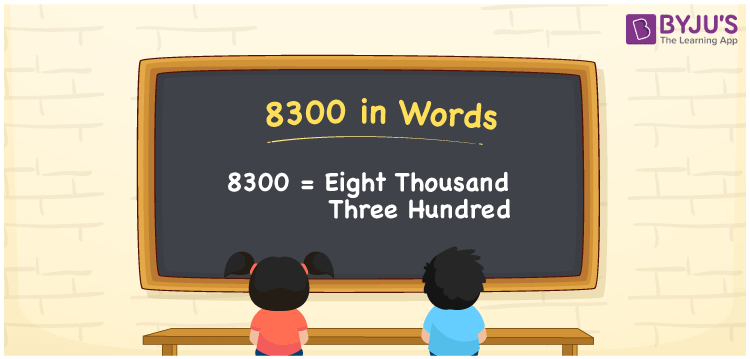# 8300 in Words

8300 in words is written as “Eight Thousand Three Hundred”. In Maths, 8300 is a cardinal number that expresses a quantity. If the price of the lamp is Rs.8300, then it can be represented as Rupees Eight Thousand Three Hundred, in English. Learn more about Numbers In Words at BYJU’S.

 8300 in Words Eight Thousand Three Hundred Eight Thousand Three Hundred in Numbers 8300

## 8300 in English Words

To make it easy for children to learn the names of the numbers we have represented the chart below. It shows the name of 8300 in English words.## How to Write 8300 in Words?

The number 8300 in words can be written using a place value system, where we can identify the position of each digit in the original number. Since 8300 is a four-digit number, thus, express the digits as:

 Thousands Hundreds Tens Ones 8 3 0 0

From the above table,

8 → Thousands place

3 → Hundreds place

0 → Tens

0 → Ones place

Hence, when we read the number from right to left, it is given by Eight Thousand Three Hundred.

### Expanded Form of 8300

We can write the expanded form as:

8 x Thousand + 3 × Hundred + 0 × Ten + 0 × One

= 8 x 1000 + 3 × 100 + 0 × 10 + 0 × 1

= 8000 + 300 + 0 + 0

= Eight Thousand Three Hundred

8300 is a whole number that is succeeded by 8299 and preceded by 8301. Learn more about the number 8300 below:

• 8300 in Words – Eight Thousand Three Hundred
• Is 8300 an odd number? – No
• Is 8300 an even number? – Yes
• Is 8300 a perfect square number? – No
• Is 8300 a perfect cube number? – No
• Is 8300 a prime number? – No
• Is 8300 a composite number? – Yes

## Frequently Asked Questions on 8300 in words

Q1

### What is 8300 in words?

8300 in words is given by Eight Thousand Three Hundred.
Q2

### What is the rule to write 8300 in words?

8300 in words is written based on the place values of each digit. 8 is at thousand place, 3 at hundred place, 0 at tens place and 0 at ones place. Thus, reading from right to left, we can express 8300 as Eight Thousand Three Hundred.
Q3

### What is the value of 8000 + 300 in words?

8000 + 300 = 8300, i.e., Eight Thousand Three Hundred in words